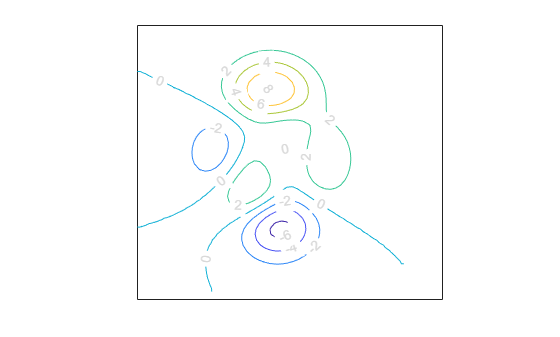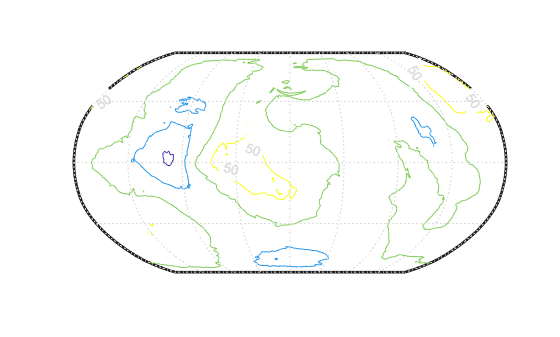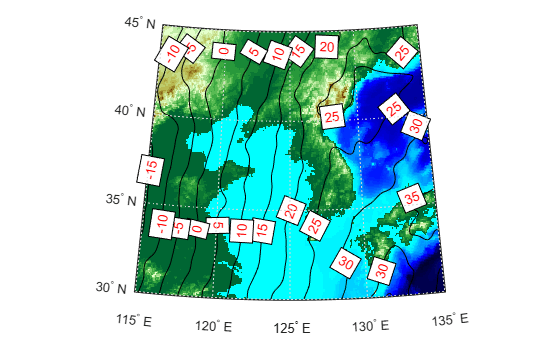# clabelm

Label map contour plot

## Syntax

``clabelm(C,h)``
``clabelm(C,h,levels)``
``clabelm(C,h,"manual")``
``t = clabelm(___)``
``clabelm(C)``
``clabelm(C,levels)``
``clabelm(C,"manual")``
``tl = clabelm(___)``
``clabelm(___,"LabelSpacing",spacing)``

## Description

### Rotated Labels

example

````clabelm(C,h)` labels the current map contour plot by inserting rotated text into each contour line. If a contour line is not long enough to fit the text, then the function does not insert the text.The `clabelm` function supports only contour plots created using the `contourfm`, `contourm`, `contour3m`, or `geoshow` function. To label other contour plots, use the `clabel` function.```

example

````clabelm(C,h,levels)` labels the contour levels specified by `levels`.```
````clabelm(C,h,"manual")` labels the locations you select with the mouse. Click the mouse or press the space bar to label the contour closest to the center of the cursor. To finish labeling, while the cursor is within the figure, press Enter.```

example

````t = clabelm(___)` returns the labels as text objects, using any combination of input arguments from the previous syntaxes in this group. Use `t` to modify properties of the text after creating it. For a list of properties, see Text Properties.```

### Upright Labels

````clabelm(C)` labels the current contour plot by adding upright text next to each contour line. The function randomly selects the positions of the labels. This syntax displays plus symbols (`+`) on the contour lines next to the labels.```
````clabelm(C,levels)` labels the contour levels specified by `levels`.```
````clabelm(C,"manual")` labels the locations you select with the mouse. Click the mouse or press the space bar to label the contour closest to the center of the cursor. To finish labeling, while the cursor is within the figure, press Enter.```
````tl = clabelm(___)` returns the labels as text objects and the plus symbols (`+`) as line objects, using any combination of input arguments from the previous syntaxes in this group.```

````clabelm(___,"LabelSpacing",spacing)` specifies the spacing between labels on the same contour line.```

## Examples

collapse all

Create a contour plot from sample map data. Obtain the contour matrix `C` and the contour patch `h`. Then, label the contour plot.

```Z = peaks; R = georefcells([-0.1 0],[0 0.1],size(Z)); axesm miller [C,h] = contourm(Z,R); clabelm(C,h)```Create a contour plot using geoid heights from the Earth Gravitational Model of 1996 (EGM96). Label only the contours with contour levels `-100` or `50`.

```[N,R] = egm96geoid; worldmap(N,R) plabel off mlabel off [C,h] = contourm(N,R,-100:50:100); clabelm(C,h,[-100 50])```Create an elevation map for a region surrounding the Korean peninsula.

```load korea5c worldmap(korea5c,korea5cR) geoshow(korea5c,korea5cR,"DisplayType","texturemap") demcmap(korea5c)```

Create a contour plot using geoid heights from the Earth Gravitational Model of 1996 (EGM96).

```N = egm96geoid(korea5cR); [C,h] = contourm(N,korea5cR,-100:5:100,"k");```

Label the contours and return the text objects. Change the text color and the edge color by setting properties of the text objects.

```t = clabelm(C,h); set(t,"Color","r") set(t,"EdgeColor","k")```## Input Arguments

collapse all

Contour matrix, specified as a two-row matrix returned by the `contourm`, `contourfm`, or `contour3m` function. This argument contains the data that defines the contour lines. The first row of the matrix contains longitude data and the second row of the matrix contains latitude data.

If you pass the contour object `h` to the `clabelm` function, then you can replace this argument with `[]`. For example, use `clabelm([],h)`.

Contour patches, specified as a `Group` object returned by the `contourm`, `contourfm`, `contour3m`, or `geoshow` function.

Contour levels, specified as a row or column vector of individual values.

Example: `[0,10,20]`

Label spacing along the contour lines, specified as a scalar value in points, where one point is 1/72 inch. Use this property to control the number of contour labels along the contour lines. Smaller values produce more labels.

If the length of a contour line is less than `spacing`, then the function displays one label on the line.

## Output Arguments

collapse all

Text objects, returned as a vector. The `UserData` properties of the text objects contain the contour values displayed.

Text and line objects, returned as a vector. The `UserData` properties of the text objects contain the contour values displayed. The line objects correspond to the plus symbols (`+`).

## Version History

Introduced before R2006a